# Inverse relationship + system of equations - math problems

#### Number of problems found: 16

• CoinsThe money - coins are minted from the hardest bronze, which contains copper and tin in a ratio of 41: 9. How much copper and tin are in 2kg of bronze money?
• Working together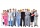Two people will do the work in 12 days. They worked together for 8 days. Then only one worked for 10 days. How many days would each of them do the work if he worked alone?
• Trio ratioHans, Alena and Thomas have a total of 740 USD. Hans and Alena split in the ratio 5: 6 and Alena and Thomas in the ratio 4: 5. How much will everyone get?
• Two diggersTwo diggers should dig a ditch. If each of them worked just one-third of the time that the other digger needs, they'd dig up a 13/18 ditch together. Find the ratio of the performance of this two diggers.
• Hyperbola equationFind the hyperbola equation with the center of S [0; 0], passing through the points: A [5; 3] B [8; -10]
• Two workersTwo workers should fulfill certain task together for 5 days. If the first worker increased their performance twice and second twice fell, it took them just four days. For how many days would handle the entire task first worker himself?
• Forth and back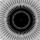Car goes from point A to point B at speed 78 km/h and back at 82 km/h. If went there and back at speed 81 km/h journey would take five minutes less. What is the distance between points A and B?
• James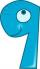James paints a fence. If every day instead of 14 planks painted 16 would be done on one day earlier. How many of planks has a fence altogether?
• Revenue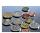Mr. Robert and Mr. Norbert get together € 1400. The revenue split in the ratio of 4:3, depending upon each work. How many got each of them?
• Three numbers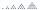What is three numbers which have the property: the sum of the reciprocals of the first and second numbers is 12/7, the first and third 11/24 and the second and the third 3/8.
• Three workers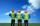Three workers A, B, C have to work on a specific task. Workers A and B completed whole task in 14 days, B together with C for 23 days, A together with C for 13 days. How long it would take to complete the task every one of them alone? How long it would ta
• Workers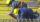Workers A and B will done work together for 15 days. They worked together 13.5 days. Then A worker became ill and worker B finish the job alone for 7.5 days. If every worker was working alone, how many days took whole work for worker A and B?
• Forestry workersIn the forest is employed 56 laborers planting trees in nurseries. For 8 hour work day would end job in 37 days. After 16 days, 9 laborers go forth? How many days are needed to complete planting trees in nurseries by others, if they will work 10 hours a d
• ExcavationMr. Billy calculated that excavation for a water connection dig for 12 days. His friend would take 10 days. Billy worked 3 days alone. Then his friend came to help and started on the other end. On what day since the beginning of excavation they met?
• Freedom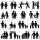In the city are 3/9 of women married for 3/6 men. What proportion of the townspeople is free (not married)? Express as a decimal number.
• PoolIf water flows into the pool by two inlets, fill the whole for 8 hours. The first inlet filled pool 6 hour longer than second. How long pool take to fill with two inlets separately?

Do you have an interesting mathematical word problem that you can't solve it? Submit a math problem, and we can try to solve it.

We will send a solution to your e-mail address. Solved examples are also published here. Please enter the e-mail correctly and check whether you don't have a full mailbox.

Please do not submit problems from current active competitions such as Mathematical Olympiad, correspondence seminars etc...

Do you have a system of equations and looking for calculator system of linear equations?